# TS Grewal Accountancy Class 11 Solution Chapter 5 Accounting Equation

Read TS Grewal Accountancy Class 11 Solution Chapter 5 Accounting Equation 2023 2024. Students should study TS Grewal Solutions Class 11 Accountancy available on Studiestoday.com with solved questions and answers. These chapter-wise answers for Class 11 Accountancy have been prepared by expert teachers of Grade 11. These TS Grewal Class 11 Solutions have been designed as per the latest accountancy TS Grewal Book for Class 11 and if practiced thoroughly can help you to score good marks in standard 11 Accounts class tests and examinations.

## Class 11 Accounts Chapter 5 Accounting Equation TS Grewal Solutions

TS Grewal Solutions for Chapter 5 Accounting Equation Class 11 Accounts have been provided below based on the latest TS Grewal Class 11 book. The answers have been prepared based on the latest 2023 2024 book for the current academic year. TS Grewal Solutions Class 11 will help students to improve their concepts and easily solve accountancy questions for Class 11. Class 11 Grewal solutions should be revised regularly as more practice will help you get a better rank and easily solve more questions.

### Chapter 5 Accounting Equation TS Grewal Class 11 Solutions

About this chapter: TS Grewal Accountancy Class 11 Chapter 5 provides details relating to Accounting Equations which are used by various organizations. It is important to understand various accounting equations which are used by organizations to better understand financial statements. It is important for students in Class 11 to understand all the accounting equations as it will help them to gain more marks in exams. Equations should be learned and practiced daily so that they can be really useful in understanding further chapters in this book. This is a very scoring chapter and if the students have practiced all questions given below then they will get higher score in examinations

Question 1. Explain in short the meaning of an Accounting Equation.

An Accounting Equation is a mathematical expression which shows that the assets and liabilities of a firm are equal. An Accounting Equation is based on the dual aspect concept of accounting meaning; every transaction has two aspects- debit and credit. It holds that for every debit there is a credit of equal amount and vice versa.

Question 2. “Accounting Equation (A=L+C) always holds good under all circumstances.” Explain with the help of two examples.

“Accounting Equation (A = L + C) always holds good under all circumstances.”

For Example,

(i) Started business with Stock = Rs. 5,500, Capital = Rs. 3,500 and Creditors = Rs. 2,000

Assets = Liabilities + Capital

Stock = Creditors + Capital

Rs. 5,500 = Rs. 2,000 + Rs. 3,500

(ii) Stock taken by the proprietor for private use Rs. 500

Assets = Liabilities + Capital

Stock = Creditors + Capital

(Rs. 5,500 – Rs. 500) = Rs. 2,000 + (Rs. 3,500 – Rs. 500)

Rs. 5,000 = Rs. 2,000 + Rs. 3,000

Rs. 5,000 = Rs. 5,000

Question 3. What is owner’s equity? Give an equation for calculating owner’s equity. Give two examples at least.

Owner’s Equity means balance standing to the credit of Capital Account of the proprietor. It is the capital investment plus earning minus expenses of the business.

The Equation to calculate Owner’s Equity or Capital = Assets – liabilities

For Example:-

(i) Started Business with Stock = Rs. 2,500, Capital = Rs. 1,500 and Creditors = Rs. 1,000

Assets = Liabilities + Capital

Owner’s Equity = Assets – Creditors

Owner’s Equity = Rs. 2,500 – Rs. 1,000

Owner’s Equity = Rs. 1,500

(ii) If the above example is continued and rent paid Rs. 500, then Owner’s Equity will be:

Owner’s Equity = Capital before rent paid – Rent paid

=Rs. 1,500 – Rs. 500

= Rs. 1000

Question 4. From the following information find the capital of Vijay: Total Assets Rs. 5,00,000; Creditors Rs. 1,00,000; Loan from Bank Rs. 1,50,000.

Capital of Vijay = Total Assets – Creditors – Loan from Bank

= Rs. 5,00,000 – Rs. 1,00,000 – Rs. 1,50,000

= Rs. 2,50,000

Question 5. If the Capital is Rs. 2,60,000 and Assets are Rs. 5,00,000, what is the amount of liabilities?

Liabilities = Total Assets – Capital

= Rs. 5,00,000 – Rs. 2,60,000

= Rs. 2,40,000

Question 6. If the Capital is Rs. 1,00,000 and Outside Liabilities are Rs. 2,50,000, find the Total Assets.

Total Assets = Outside Liabilities + Capital

= Rs. 2,50,000 + Rs. 1,00,000

= Rs. 3,50,000

Question.7. Ram started business on 1st April, 2020 with a Capital of Rs. 25,000 and a loan of Rs. 12,500. On 31st March, 2021, his assets were Rs. 50,000. Find his capital as on 31st March, 2021 and the profit earned during the year.

Capital of Ram on 31st March, Current Year= Total Assets – Loan

= Rs. 50,000 – Rs. 12,500

= Rs. 37,500

Profit of Ram for the year ended 31st March (Current Year)

= Capital on 31st March (Current year) – Capital on 1st April (Last Year)

= Rs. 37,500 – Rs. 25,000

= Rs. 12,500

Question 8. Calculate total assets if:

Capital is Rs. 2,00,000; Creditors Rs. 50,000; Revenue during the year Rs. 5,00,000; and Expenses during the year Rs. 4,00,000.

Total Assets = Creditors + Capital

= Creditors + (Capital + Revenue – Expenses)

= Rs. 50,000 + (Rs. 2,00,000 + Rs. 5,00,000 – Rs. 4,00,000)

= Rs. 50,000 + Rs. 3,00,000

= Rs. 3,50,000

Question 9. (a) A starts a business and invests Rs. 50,000 on 1st April, 2020. On 31st March, 2019 his assets are Rs. 65,000 and liabilities are Rs. 6,000. Find out the amount of capital on 31st March,2021 and his profit.

(b) In the above case, if the proprietor had invested Rs. 5,000 as additional capital and withdrawn Rs. 2,000, what will be your answer?

(c) If a had withdrawn from his business Rs. 3,000 for personal use, find out the profit in this case.

(a) Closing Capital = Assets – Liabilities

= Rs. 65,000 – Rs. 6,000

= Rs. 59,000

Profit = Closing Capital – Opening Capital

= Rs. 59,000 – Rs. 50,000

(b) Profit = Closing Capital – Opening Capital – Additional Capital + Drawings

= Rs. 59,000 – Rs. 50,000 – Rs. 5,000 + Rs. 2,000

= Rs. 6,000

(c) Profit = Closing Capital – Opening Capital + Drawings

= Rs. 59,000 – Rs. 50,000 + Rs. 3,000

= Rs. 12,000

Question 10. Determine the missing amounts on the basis of the Accounting Equation: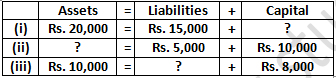The missing amounts were determined on the basis of the following Accounting Equation: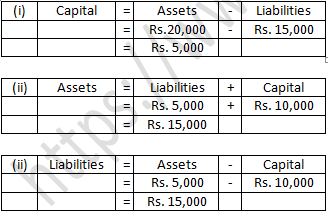Question 11. The assets of a business on 31st March, 2019 are Rs. 50,000 and its capital is Rs. 35,000. Calculate its liabilities on that date.(Old Question)

The Liabilities of the firm as on 31st March of the Current Year = Assets – Capital

= Rs. 50,000 – Rs. 20,000

= Rs. 30,000

Practical Problems  --->>

Question 1:  What will be effect of the following on the Accounting Equation?

(i) Started business with cash Rs 45,000
(ii) Opened a Bank Account with a deposit of Rs 4,500
(iii) Bought goods from M\s. Sun & Co. For Rs11,200Points for Knowledge:-

• In (iii) the name of the seller is given so it will be treated as credit sale. It will create effect on the liability side (creditors) also.

Question 2:  Show the Accounting Equation for the following transactions: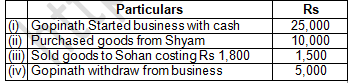Points for Knowledge:-

• In (ii) transaction because the name of the seller is given so it will be treated as credit sale. It will create effect on liability side (creditors) also.
• In (iv) Gopinath who is the proprietor of the business takes out Rs.5, 000 from Business.

Question 3:   Prepare an Accounting Equation and Balance Sheet on the following basis:

(i)  Ajeet started business Rs 20,000.
(ii) He purchased furniture for Rs 2,000.
(iii) He paid rent of Rs 200.
(iv) He purchase goods on credit Rs 3,000.
(v) He sold goods (cost price Rs 2,000) for Rs 5,000 on cash.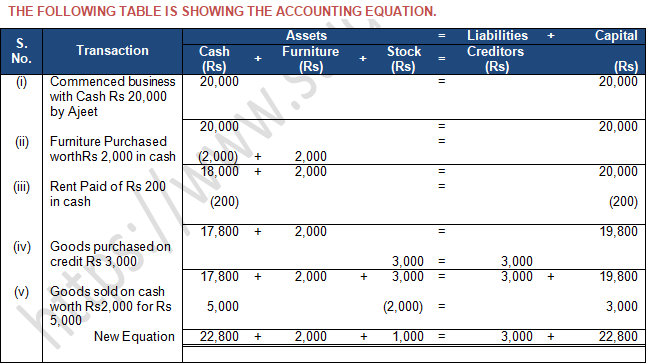Points of Knowledge:-

• If the question is silent, we assume that sales and purchases are made in cash mode.

Question 4:  Prepare Accounting Equation from the following:

(i)  Started business with cash Rs 1,00,000 and Goods Rs 20,000.
(ii) Sold goods worth Rs 10,000 for cash Rs 12,000.
(iii) Purchased furniture on credit for Rs 30,000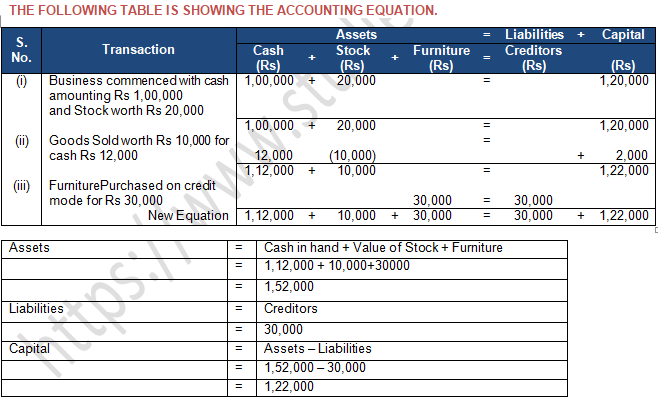Question 5:  Develop an Accounting Equation from the following transactions: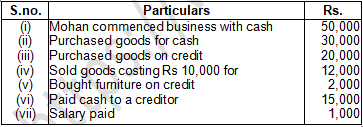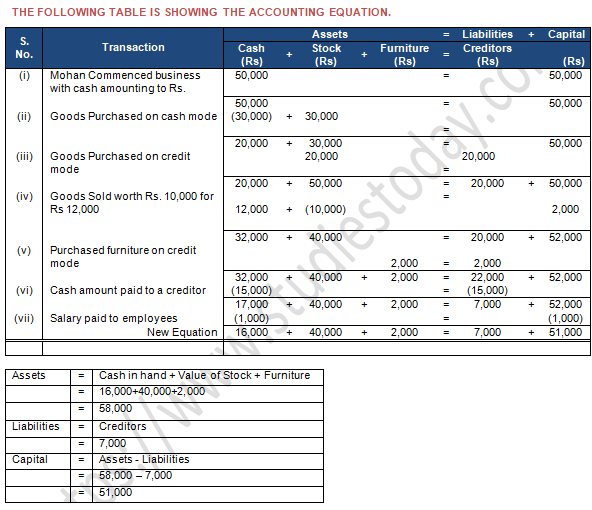Question.6: Use accounting equation to show off effect of the following transactions of M/s Royal Traders:

(a) Started a Business with Cash Rs. 1,20,000.

(b) Purchased goods for Cash Rs. 10,000.

(d) Salary outstanding Rs. 2,000.

(e) Sold goods for cash (Costing Rs. 5,000) Rs. 7,000.

(f) Goods destroyed by fire Rs. 500.Question 7:  Show the effect of the following transactions on the Accounting Equation:

(i) Started business with cash Rs 50,000.
(ii) Salaries paid Rs 2,000.
(iii) Wages Outstanding Rs 200.
(iv) Interest due but not paid Rs 100.
(v) Rent paid in advance Rs 150.Points for Knowledge:-

• Outstanding expenses (iii) are always liability for a business.
• Rent paid in advance (v) is an asset as it is prepaid in Nature.

Question 7:  Prepare an Accounting Equation from the following:      (Old Question)

(i) Started business with cash Rs 1,00,000.
(ii) Purchased goods for cash Rs 20,000 and on credit Rs 30,000.
(iii) Sold goods for cash costing Rs 10,000 and on credit costing Rs 15,000 both at a profit of 20%.Points of Knowledge:-

• In (ii) Calculation of total goods purchased Rs. 20,000(cash) + Rs.30, 000(credit) i.e, 50,000 in total.

Question 8:  What will be the effect of the following on the Accounting Equation?

(i) Harish started business with cash Rs 18,000
(ii) Purchased goods for CashRs5,000 and on credit Rs 2,000
(iii) Sold goods for cash Rs 4,000 (costing Rs 2,400)
(iv) Rent paid  Rs1,000 and Rent Outstanding Rs 200Points for Knowledge:-

• Total Stock Purchases (ii) by Harish is 5,000 (Cash) + 2,000 (Credit) i.e., 7,000 in total.
• Outstanding rent (iv), as outstanding in nature, so it is always liability for a business.

Question 9:   Prepare an Accounting Equation and Balance Sheet on the following basis:

(i)  Ajeet started business Rs 20,000.
(ii) He purchased furniture for Rs 2,000.
(iii) He paid rent of Rs 200.
(iv) He purchase goods on credit Rs 3,000.
(v) He sold goods (cost price Rs 2,000) for Rs 5,000 on cash.Points of Knowledge:-

• If the question is silent, we assume that sales and purchases are made in cash mode.

Question 10:  Prove that the Accounting Equation is satisfied in all the following transactions of Suresh. Also prepare a Balance Sheet.

(i) Commenced business with cash Rs 60,000.

(ii) Paid Rent in Advance Rs 500.

(iii) Purchased goods for Cash Rs 30,000 and Credit Rs 20,000.

(iv) Sold goods for Cash Rs 30,000 Costing Rs 20,000.

(v) Paid Salary Rs 500 and Salary Outstanding being Rs 100.

(vi) Bought motorcycle for personal use Rs5,000.Points of Knowledge:-

• In (vi) motorcycle purchased for personal use so it is treated as drawing, it is subtracted from capital. Anything purchased for personal use should be treated as drawing.

Question 11:  Show the effect of the following transactions on assets, liabilities and capital using the Accounting Equation. Also prepare a Balance Sheet.

(i) Started business with Cash Rs 60,000
(iii) Accrued Interest Rs 500
(v) Amount withdrawn Rs 5,000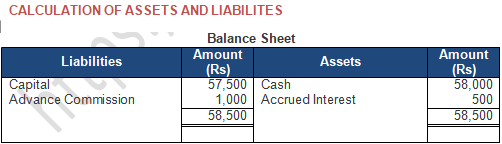Points of Knowledge:-

• In (iii) accrued interest is an asset for business. Hence, shown on the asset side.

Question 12:  Raghunath had the following transactions in an accounting year:

(i) Commenced business with cash Rs50,000.

(ii) Paid in to bank Rs 10,000.

(iii) Purchased goods for Cash Rs 20,000 and Credit Rs 30,000.

(iv) Sold goods for Cash Rs 40,000 Costing Rs 30,000.

(v) Rent paid Rs 500.

(vi) Rent Outstanding Rs 100.

(vii) Bought furniture Rs 5,000 on credit.

(viii) Bought refrigerator for personal use Rs 5,000.

(ix) Purchased motorcycle for cash Rs 20,000.

Create an Accounting Equations to show the effect of the above transaction on his assets, liabilities and capital and also show his final Balance Sheet.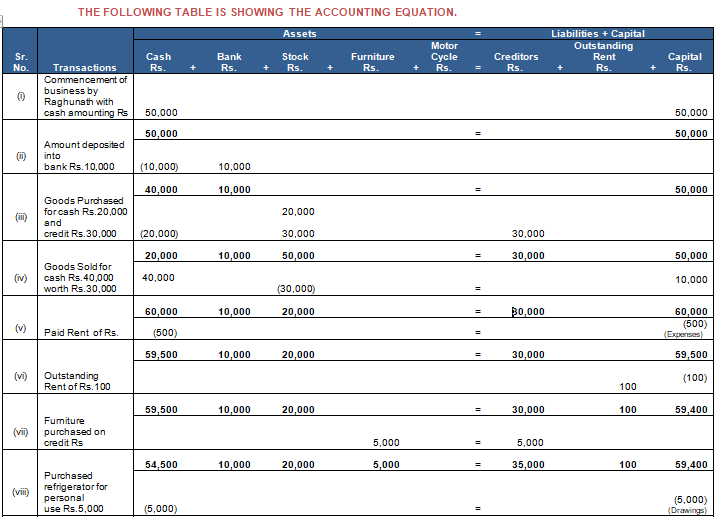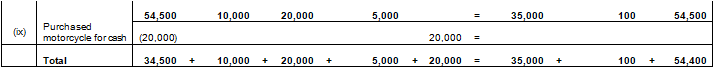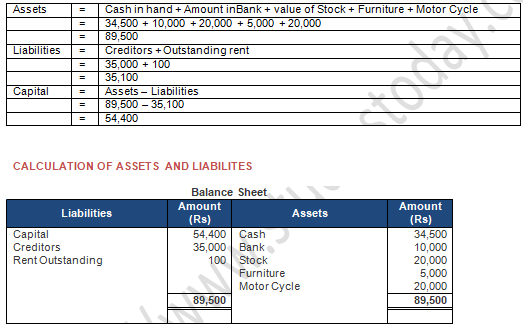Points of Knowledge:-

• In (ii) Calculation of total goods purchased Rs. 20,000(cash) + Rs.30,000 (credit) i.e.50,000 in total.
• In(vi) Here Refrigerator purchased by Raghunath for personal use so it is treated as drawing. It is subtracted from capital.

Question 12:  Prove that the Accounting Equation is satisfied in all the following transactions of Sameer Goel:(Old Question)

(i) Started business with cash Rs 10,000.

(ii) Paid rent in Advance Rs 300.

(iii) Purchased goods for cash Rs 5,000 and credit Rs 2,000.

(iv) Sold goods for cash Rs 8,000 costing Rs 4,000.

(v)  Paid salary Rs 450 and salary outstanding being Rs100.

(vi) Bought motorcycle for personal use Rs 3,000.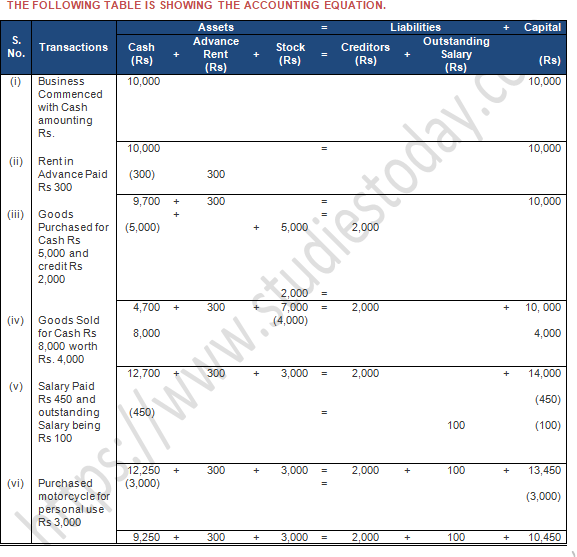Points of Knowledge:-

• In (ii) Calculation of total goods purchased Rs. 5,000(cash) + Rs.2, 000 (credit) i.e., 7,000 in total.
• In (vi) Here motorcycle purchased for personal use so it is treated as drawing, it is subtracted from capital.

Question 13:  Prepare an Accounting Equation from the following :

(i) Started business with cash Rs 50,000 and goods Rs 30,000.
(ii) Purchased goods for cash Rs 30,000 and on credit from Karan Rs 20,000.
(iii) Goods costing Rs 40,000 were Sold for Rs 55,000.
(iv) Withdrew cash for personal use Rs 10,000.
(v) Rent outstanding Rs 2,000.Question 14:  Prepare Accounting Equation from thefollowing :

(a) Started business with cash Rs 1,00,000.

(b) Purchase goods for cash Rs 20,000 and on credit Rs 30,000.

(c) Sold goods for cash costing Rs 10,000 and on credit costing Rs15,000 both at a profit of 20%.

(d) Paid salaries Rs 8,000.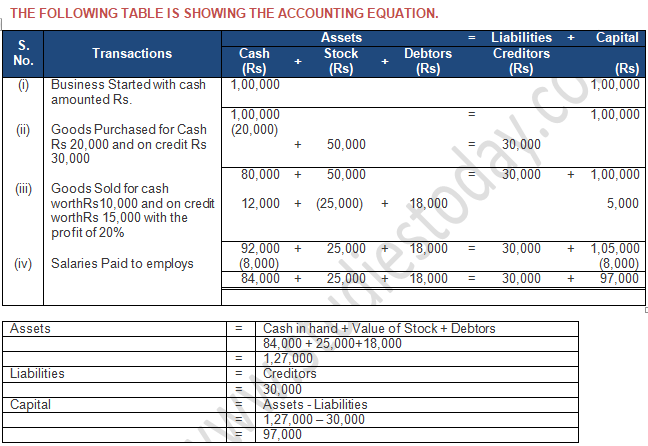Points of Knowledge:-

• Total goods purchases = Cash Purchases + Credit Purchases

=  20,000 + 30,000 = 50,000

• Calculation of profit on sale = Cash Sale × % of profit

= 10,000 × 20% = 2,000

= Credit Sale × % of Profit

= 15,000 × 20% = 3,000

= Total Profit = 2,000+3,000

Question 15:  Show an Accounting Equation for the following transactions:

(i) D. Mahapatra commenced business with cash of Rs 50,000 and Rs 1,00,000 by cheque; goods Rs 60,000; machinery Rs 1,00,000 and furniture Rs 50,000.

(ii) 1/3rd of above goods sold at a profit of 10% on cost, and half of the payment is received in cash.

(iii) Depreciation on machinery provided @10%.

(iv) Cash withdrawn for personal use Rs 10,000.

(v) Interest on drawings charged @ 5%.

(vi) Goods Sold to Gupta for Rs 10,000 and received a Bill Receivable for the same amount for 3 months.

(vii) Received Rs 10,000 from Gupta against the Bill Receivable on its maturity.Points of knowledge:-

• Total Capital= Cash + Bank + Stock + Machinery + Furniture

50,000 + 1, 00,000 + 60,000 + 1,00,000 + 50,000 = 3,60,000

• Total Value of stock = Rs. 60,000

Value of  1/3 rd Stock = 60,000 × 1/3   = Rs. 20,000

Calculation of Profit = 20,000 × 10% = Rs. 2,000

Total Sales= Value of stock+ Profit

20,000 + 2,000= Rs. 22,000

Half of total sales value received in cash = 22000 × ½ =Rs. 11,000

• Interest on drawings = 10,000 × 5% = Rs. 500

Question 16:  Show an Accounting Equation on the basis of the following transactions: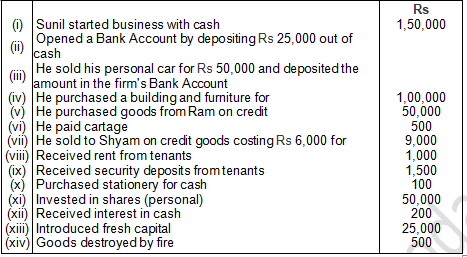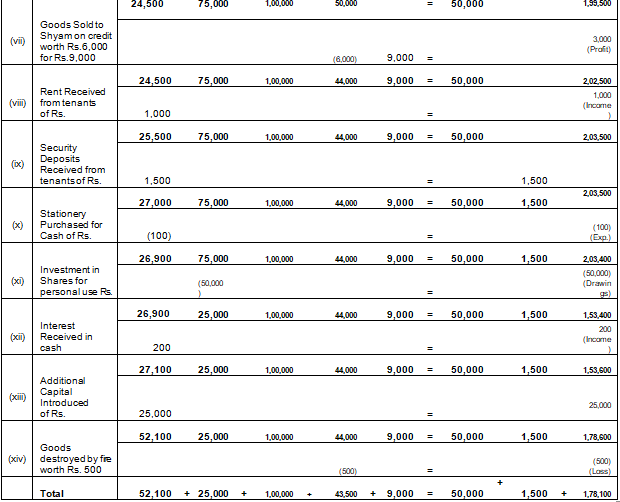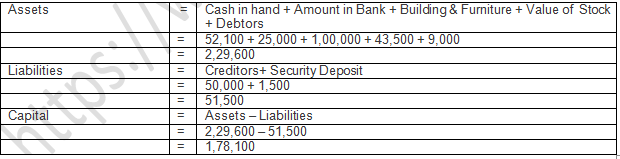Points of knowledge: -

• In (vi) cost of Building & Furniture is Rs. 1,00,000. Don’t Separate it.
• In (xi) Investment in share market for personal use should be treated as drawings.

Question 17:  If the capital of a business is Rs 3,00,000 and liabilities are Rs 50,000, loss Rs 70,000, calculate the total assets of the business.TOTAL ASSETS WILL BE RS. 2,80,000

Question 17:  Show the accounting equation on the basis of following transactions:(Old Question)

(a) Ram started business with Rs 25,000.

(b) Purchased goods from Shyam Rs 10,000.

(c) Sold goods to Sohan costing Rs 1,500 for Rs 1,800.Points for Knowledge:-

• In (ii) The name of the seller is given so it will be treated as credit sale. It will create effect on liability side (creditors) also.

Question 18:  If total assets of a business are Rs 1,30,000 and Net worth is Rs 80,000, calculate the creditors .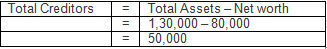TOTAL CREDITORS WILL BE RS. 2,80,000

Question 19:  A commenced his cloth business on 1st April, 2017 with a capital of Rs 30,000. On 31st March 2018, his assets were worth Rs 50,000 and liabilities of Rs10,000. Find out his closing capital and profits earned during the year.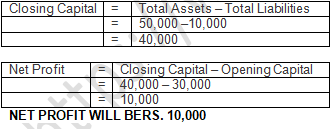Question 20:  If capital of a business is Rs 1,40,000 and liabilities are of Rs 80,000, calculate the total assets of the business.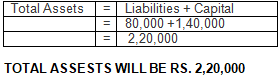Question 21:  Calculate the total assets if:

(i) Capital is Rs 40,000.
(ii) Creditors are Rs 25,000.
(iii) Revenue during the period is Rs 50,000.
(iv) Expenses during the period are Rs 40,000.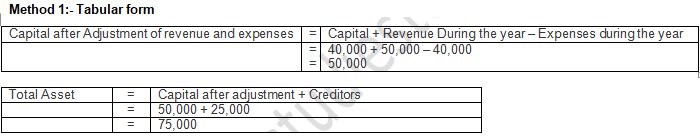Method 2:-

Total assets = Opening capital + Revenue during the year – Expenses during the year + creditors

= 40,000 + 50,000 – 40,000 + 25,000

= 75,000

Question 22:

(a) A had a capital of Rs 75,000 on 1st April, 2017. He had also goods amounting to Rs 15,000 which he had purchased on credit and the payment had not been made. Find out the value of the total assets of the business.

(b) After a period of one month, he came to know that he had suffered a loss of Rs 1,700. He withdrew Rs 800 for his personal use. Find out his capital and assets of the business.Question 23:

(a) Mohan started a business on 1st April, 2017 with a capital of Rs 10,000 and borrowed Rs 3,000 form a friend . He earned a profit of Rs 5,000 during the year ended 31st March, 2018 and withdrew cash Rs 4,000 for private use. What is his capital on 31st March, 2018?

(b) Mahesh started a business with a capital of Rs 15,000 on 1st April, 2017. During the year, he made a profit of Rs 3,000. He owes Rs 2,500 to suppliers of goods . What is the total of assets in his business on 31st March, 2018?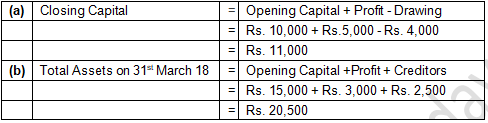Question 24:

On 31st March, 2019, the total assets and external liabilities were Rs 2,00,000 and Rs 6,000 respectively . During the year, the proprietor had introduced capital of Rs 20,000 and withdrawn Rs 12,000 for personal use. He made a profit of Rs 20,000 during the year. Calculate the capital as on 1st April, 2018CAPITAL AS ON MARCH 31, 2018 = 1,94,000

CAPITAL ON APRIL 01, 2017 = 1,66,000

Question 25:  Mohan started a business on 1st April, 2017 with a capital of Rs 25,000 and a loan of Rs 12,500 borrowed from Shyam. During 2018-19 he had introduced additional capital of 12,500 and had withdrawn Rs 7,500 for personal use. On 31st March, 2019 his assets were Rs 75,000. Find out his capital as on 31st March, 2019 and profit made or loss incurred during the year 2018-19.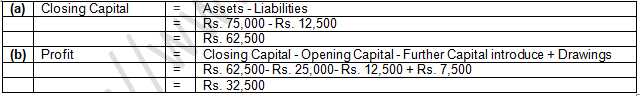PROFIT DURING THE YEAR 2017-18 = 32,500

Question 26: Calculate the amount of External Equities as on 31st March, 2021 in the following cases:

(i) On 31st March, 2021, total assets and capital were Rs. 15,00,000 and Rs. 10,50,000 respectively.

(ii) On 1st April, 2020, Mukesh started business with a capital of Rs. 3,00,000 and a loan of Rs. 3,00,000 borrowed from a friend. During the year, he earned a profit of Rs. 1,50,000. On 31st March, 2021 the total assets were Rs. 15,00,000.

(iii) On 1st April, 2020, Ramesh started business with a capital of Rs. 3,00,000 and  a loan of Rs. 3,00,000 borrowed from a fixed. During the year, he earned a profit of Rs. 1,50,000, introduced an additional capital of Rs. 1,80,000 and had withdrawn Rs. 90,000 for his personal use. On 31st March, 2021 total assets were Rs. 15,00,000.

(i) External Liabilities = Assets – Capital

External Liabilities = Rs. 15,00,000 – Rs. 10,50,000

External Liabilities = Rs. 4,50,000

(ii) Closing Capital = Opening Capital + Additional Capital + Profit – Drawings

Closing Capital = Rs. 3,00,000 + Rs. 0 + Rs. 1,50,000 – Rs. 0

Closing Capital = Rs. 4,50,000

External Liabilities = Assets – Capital

External Liabilities = Rs. 15,00,000 – Rs. 4,50,000

External Liabilities = Rs. 10,50,000

(iii) Closing Capital = Opening Capital + Additional Capital + Profit – Drawings

Closing Capital = Rs. 3,00,000 + Rs. 1,80,000 + Rs. 1,50,000 – Rs. 90,000

Closing Capital = Rs. 5,40,000

External Liabilities = Assets – Capital

External Liabilities = Rs. 15,00,000 – Rs. 5,40,000

External Liabilities = Rs. 9,60,000

 DK Goel Solutions Class 11 Accountancy Chapter 1 Meaning and Objective of Accounting DK Goel Solutions Class 11 Accountancy Chapter 2 Basic Accounting Terms DK Goel Solutions Class 11 Accountancy Chapter 3 Accounting Principles DK Goel Solutions Class 11 Accountancy Chapter 4 Process and Bases of Accounting DK Goel Solutions Class 11 Accountancy Chapter 5 Accounting Standards and International Financial Reporting Standards DK Goel Solutions Class 11 Accountancy Chapter 6 Accounting Equations DK Goel Solutions Class 11 Accountancy Chapter 7 Double Entry System DK Goel Solutions Class 11 Accountancy Chapter 8 Origin of Transactions Source Documents of Accountancy DK Goel Solutions Class 11 Accountancy Chapter 9 Books of Original Entry Journal DK Goel Solutions Class 11 Accountancy Chapter 10 Accounting for Goods and Service Tax DK Goel Solutions Class 11 Accountancy Chapter 11 Books of Original Entry Cash Book DK Goel Solutions Class 11 Accountancy Chapter 12 Books of Original Entry Special Purpose Subsidiary Books DK Goel Solutions Class 11 Accountancy Chapter 13 Ledger DK Goel Solutions Class 11 Accountancy Chapter 14 Trial Balance and Errors DK Goel Solutions Class 11 Accountancy Chapter 15 Bank Reconciliation Statement DK Goel Solutions Class 11 Accountancy Chapter 16 Depreciation DK Goel Solutions Class 11 Accountancy Chapter 17 Provision and Reserves DK Goel Solutions Class 11 Accountancy Chapter 18 Bills of Exchange DK Goel Solutions Class 11 Accountancy Chapter 19 Rectification of Errors DK Goel Solutions Class 11 Accountancy Chapter 20 Capital and Revenue DK Goel Solutions Class 11 Accountancy Chapter 21 Financial Statement DK Goel Solutions Class 11 Accountancy Chapter 22 Financial Statements With Adjustments DK Goel Solutions Class 11 Accountancy Chapter 23 Accounts from Incomplete Records DK Goel Solutions Class 11 Accountancy Chapter 24 Introduction to Computer DK Goel Solutions Class 11 Accountancy Chapter 25 Introduction of Accounting Information System DK Goel Solutions Class 11 Accountancy Chapter 26 Computerised Accounting System DK Goel Solutions Class 11 Accountancy Chapter 27 Accounting Software Package Tally
 TS Grewal Accountancy Class 11 Solution Chapter 1 Introduction of Accounting TS Grewal Accountancy Class 11 Solution Chapter 2 Basic Accounting Terms TS Grewal Accountancy Class 11 Solution Chapter 3 Accounting Standards and IFRS TS Grewal Accountancy Class 11 Solution Chapter 4 Bases of Accounting TS Grewal Accountancy Class 11 Solution Chapter 5 Accounting Equation TS Grewal Accountancy Class 11 Solution Chapter 6 Accounting Procedures Rules of Debit and Credit TS Grewal Accountancy Class 11 Solution Chapter 7 Origin of Transactions Source Documents and Preparation of Voucher TS Grewal Accountancy Class 11 Solution Chapter 8 Journal TS Grewal Accountancy Class 11 Solution Chapter 9 Ledger TS Grewal Accountancy Class 11 Solution Chapter 10 Special Purpose Books I Cash Book TS Grewal Accountancy Class 11 Solution Chapter 11 Special Purpose Books II Other Book TS Grewal Accountancy Class 11 Solution Chapter 12 Accounting of Goods and Services Tax (GST) TS Grewal Accountancy Class 11 Solution Chapter 12 Bank Reconciliation Statement TS Grewal Accountancy Class 11 Solution Chapter 13 Trial Balance TS Grewal Accountancy Class 11 Solution Chapter 14 Depreciation TS Grewal Accountancy Class 11 Solution Chapter 15 Provisions and Reserves TS Grewal Accountancy Class 11 Solution Chapter 16 Accounting for Bills of Exchange TS Grewal Accountancy Class 11 Solution Chapter 17 Rectification of Errors TS Grewal Accountancy Class 11 Solution Chapter 18 Financial Statements of Sole Proprietorship TS Grewal Accountancy Class 11 Solution Chapter 19 Adjustments in Preparation of Financial Statements TS Grewal Accountancy Class 11 Solution Chapter 20 Accounts from Incomplete Records Single Entry System TS Grewal Accountancy Class 11 Solution Chapter 21 Computers in Accounting TS Grewal Accountancy Class 11 Solution Chapter 22 Accounting Software Tally

Tags